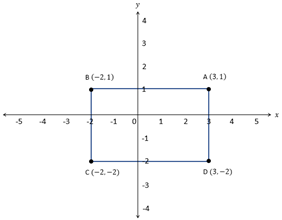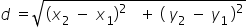Mathematics
Easy

Question

# Find the perimeter of rectangle ABCD.## 10    14    16    8Hint:

## The correct answer is: 16

### The given coordinates of the corner are as followsA = (3,1)B = (-2,1)C = (-2,-2)D = (3, -2)Now, we have to find the length of each side.Length means we have to find the distance between each points. To find the distance between the points, we will use this distance formula.Let’s find the sides one by one Side AB has two points A and B. We will find the distance between them.          .So, the distance of AB is 5 unitsSide BC has two points B and C. We will find the distance between them.So, the distance of BC is 3 units.Side CD has two points C and D. We will find the distance between themSo, the distance CD is 5 unitsSide DA has two points D and A. We will find distance between them.So, the distance DA is 3 units.Now we have all fours sides. So, to find the perimeter, we just have to add the sides.Perimeter of ABCD = AB + BC + CD + DA= 5 + 3 + 5 + 3= 16 unitsTherefore, perimeter of given rectangle is 16 units.

Instead of finding all four sides, we can just find any two adjacent sides. We can use the forumula of perimeter of rectangle 2(l + b). This distance formula is more useful when the sides of rectangle are not exactly parallel to the coordination axis. We can find length is simpler way as one of the component is zero. Say side AB is parallel to y axis, so it's y component is zero.

### Related Questions to study#### With Turito Foundation.#### Get an Expert Advice From Turito.